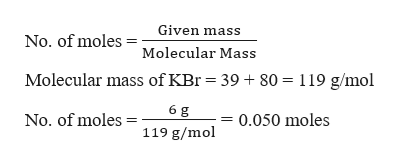# when a 6.00 g sample of KBr is dissolved in water in calorimeter that has a total heat capacity of 3.24kJ x K^-1, the temperature decreases by 0.310 K. Calculate the molar heat of solution of KBr.

Question
129 views

when a 6.00 g sample of KBr is dissolved in water in calorimeter that has a total heat capacity of 3.24kJ x K^-1, the temperature decreases by 0.310 K. Calculate the molar heat of solution of KBr.

check_circle

Step 1

The heat of the reaction when 6 g of KBr is dissolved can be calculated as,

Step 2

Molar heat of solution is the heat produced by 1 mole of the sample. Hence the number of moles of KBr in 6 g is calculated as,help_outlineImage TranscriptioncloseGiven mass No. of moles Molecular Mass Molecular mass of KBr = 39 +80 = 119 g/mol 6 g 0.050 moles No. of moles 119 g/mol fullscreen
Step 3

Now, 0.050 moles of KBr produces 1.0044 kJ of heat, hence the ...

### Want to see the full answer?

See Solution

#### Want to see this answer and more?

Solutions are written by subject experts who are available 24/7. Questions are typically answered within 1 hour.*

See Solution
*Response times may vary by subject and question.
Tagged in

### Chemical Thermodynamics